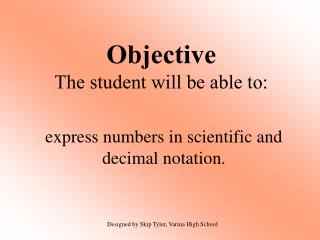DownloadDownload PresentationObjective The student will be able to:

# Objective The student will be able to:

Télécharger la présentation## Objective The student will be able to:

- - - - - - - - - - - - - - - - - - - - - - - - - - - E N D - - - - - - - - - - - - - - - - - - - - - - - - - - -
##### Presentation Transcript

1. ObjectiveThe student will be able to: express numbers in scientific and decimal notation. Designed by Skip Tyler, Varina High School

2. How wide is our universe? 210,000,000,000,000,000,000,000 miles (22 zeros) This number is written in decimal notation. When numbers get this large, it is easier to write them in scientific notation.

3. Scientific Notation A number is expressed in scientific notation when it is in the form a x 10n where a is between 1 and 10 and n is an integer

4. Write the width of the universe in scientific notation. 210,000,000,000,000,000,000,000 miles Where is the decimal point now? After the last zero. Where would you put the decimal to make this number be between 1 and 10? Between the 2 and the 1

5. 2.10,000,000,000,000,000,000,000. How many decimal places did you move the decimal? 23 When the original number is more than 1, the exponent is positive. The answer in scientific notation is 2.1 x 1023

6. 1) Express 0.0000000902 in scientific notation. Where would the decimal go to make the number be between 1 and 10? 9.02 The decimal was moved how many places? 8 When the original number is less than 1, the exponent is negative. 9.02 x 10-8

7. Write 28750.9 in scientific notation. • 2.87509 x 10-5 • 2.87509 x 10-4 • 2.87509 x 104 • 2.87509 x 105

8. 2) Express 1.8 x 10-4 in decimal notation. 0.00018 3) Express 4.58 x 106 in decimal notation. 4,580,000 On the graphing calculator, scientific notation is done with the button. 4.58 x 106 is typed 4.58 6

9. 4) Use a calculator to evaluate: 4.5 x 10-5 1.6 x 10-2 Type 4.5 -5 1.6 -2 You must include parentheses if you don’t use those buttons!! (4.5 x 10 -5) (1.6 x 10 -2) 0.0028125 Write in scientific notation. 2.8125 x 10-3

10. 5) Use a calculator to evaluate: 7.2 x 10-9 1.2 x 102On the calculator, the answer is: 6.E -11 The answer in scientific notation is 6 x 10 -11 The answer in decimal notation is 0.00000000006

11. 6) Use a calculator to evaluate (0.0042)(330,000).On the calculator, the answer is 1386. The answer in decimal notation is 1386 The answer in scientific notation is 1.386 x 103

12. 7) Use a calculator to evaluate (3,600,000,000)(23).On the calculator, the answer is: 8.28 E +10 The answer in scientific notation is 8.28 x 10 10 The answer in decimal notation is 82,800,000,000

13. Write (2.8 x 103)(5.1 x 10-7) in scientific notation. • 14.28 x 10-4 • 1.428 x 10-3 • 14.28 x 1010 • 1.428 x 1011

14. Write in PROPER scientific notation.(Notice the number is not between 1 and 10) 8) 234.6 x 109 2.346 x 1011 9) 0.0642 x 104 on calculator: 642 6.42 x 10 2

15. Write 531.42 x 105 in scientific notation. • .53142 x 102 • 5.3142 x 103 • 53.142 x 104 • 531.42 x 105 • 53.142 x 106 • 5.3142 x 107 • .53142 x 108